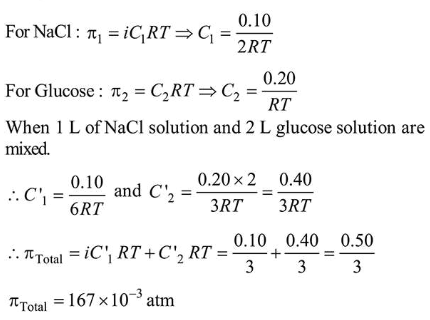# The osmotic pressure of a solution of NaCl is 0.10 atm and that of a glucose solution is 0.20 atm.Question:

The osmotic pressure of a solution of $\mathrm{NaCl}$ is $0.10 \mathrm{~atm}$ and that of a glucose solution is $0.20$ atm. The osmotic pressure of a solution formed by mixing $1 \mathrm{~L}$ of the sodium chloride solution with $2 \mathrm{~L}$ of the glucose solution is $x \times 10^{-3}$ atm. $x$ is_________________ (nearest integer)

Solution:

(167)